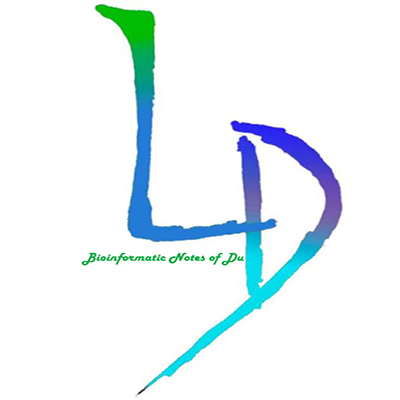V1

2023/05/18阅读：13主题：全栈蓝

# 跟着Bioinformatics学作图 | Seqlogo图绘制

-- Du

## Seqlogo图

### 下载流程

1. Step one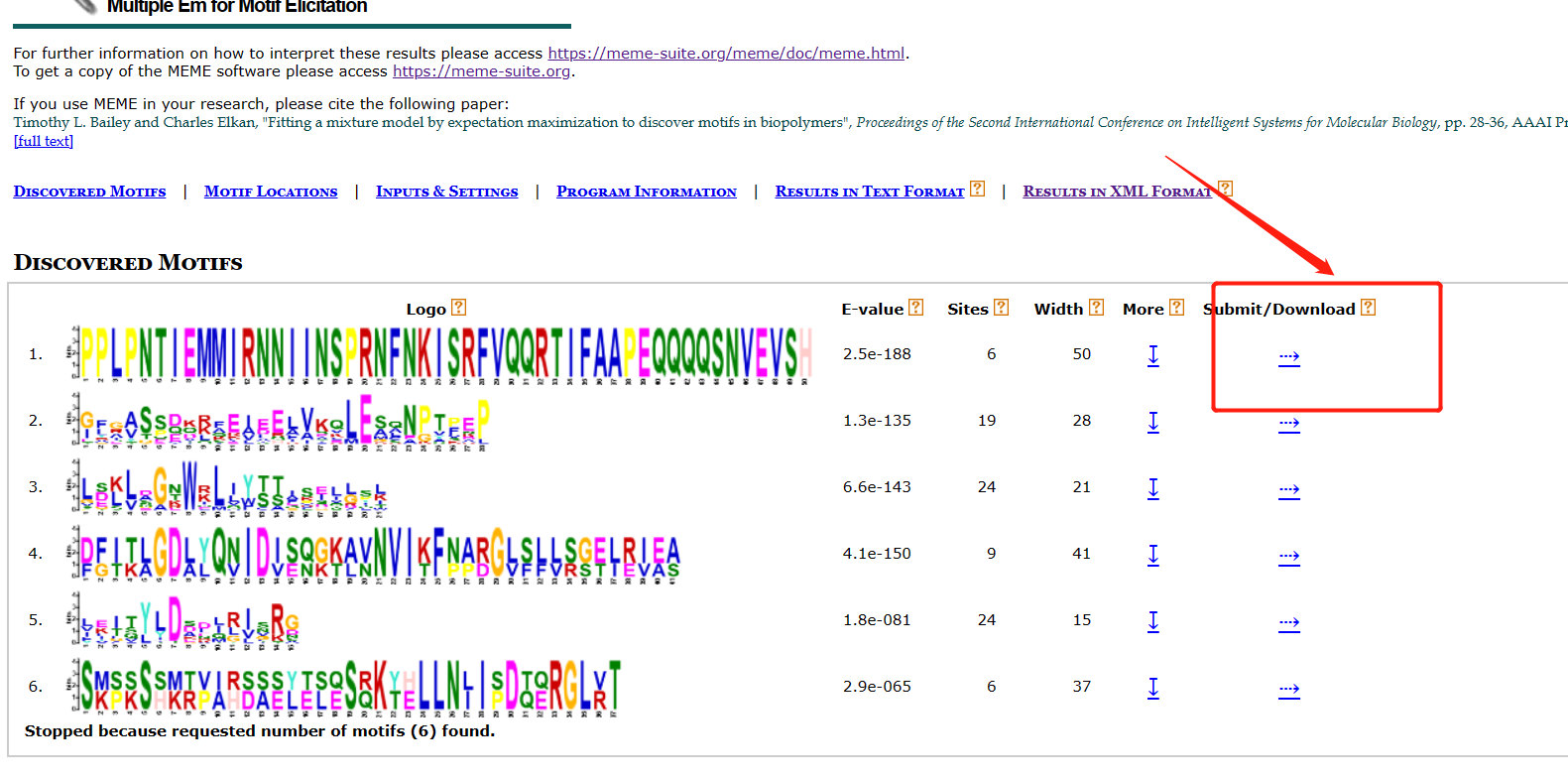2. Step Two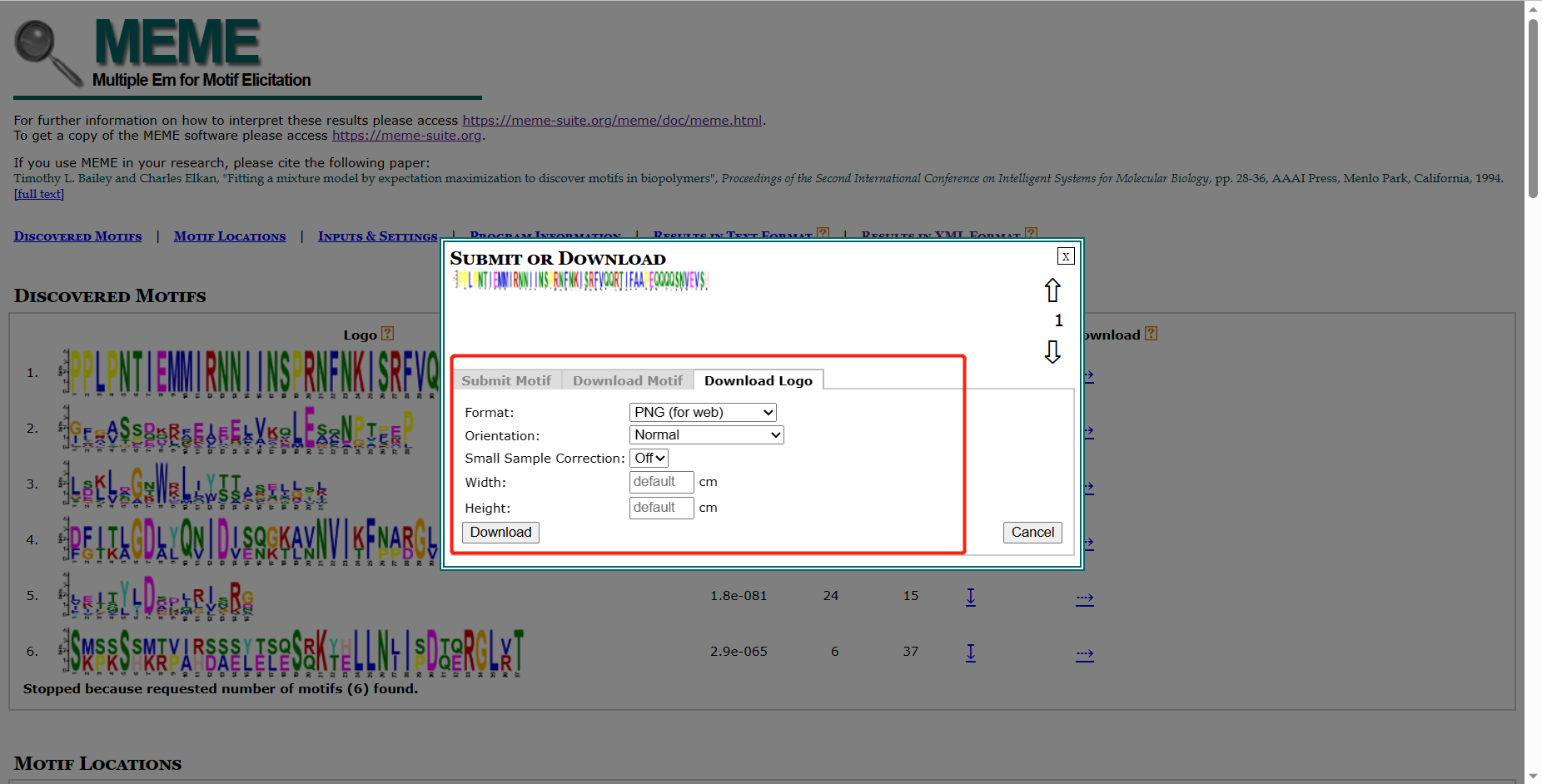3. ENDING !

## 基于ggseqlogo包绘制

ggseqlogo包发表在Bioinformatic期刊中，使用相对简洁、方便，但是感觉批量绘制时颜色很难修改(PS:应该自己参数没使用对)。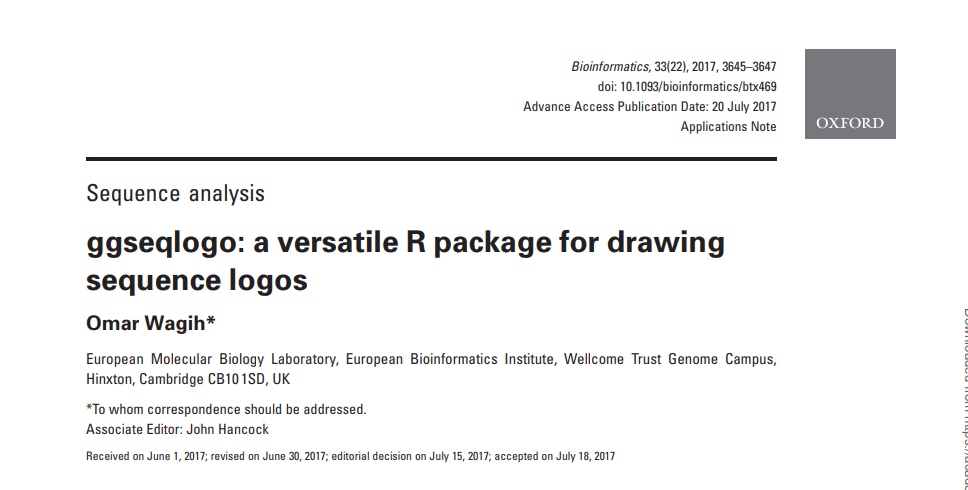文章网址：

``https://academic.oup.com/bioinformatics/article/33/22/3645/3980251?login=false``

ggseqlogo包使用网址：

``https://omarwagih.github.io/ggseqlogo/``

### 安装R包

``install.packages("ggseqlogo")or devtools::install_github("omarwagih/ggseqlogo")``

### 加载所需包

``# Load the required packagesrequire(ggplot2)require(ggseqlogo)``

``·## 批量生产文件名filelist = c(paste0('motif',1:10,'.txt'))filelen <- length(filelist)##批量读取data.list <- list()for (i in 1:10) {  data.list[[paste0('motif',i)]]=scan(filelist[i],what = '')}``

``# Some sample datadata(ggseqlogo_sample)``

``ggplot() + geom_logo( seqs_dna\$MA0001.1 ) + theme_logo()``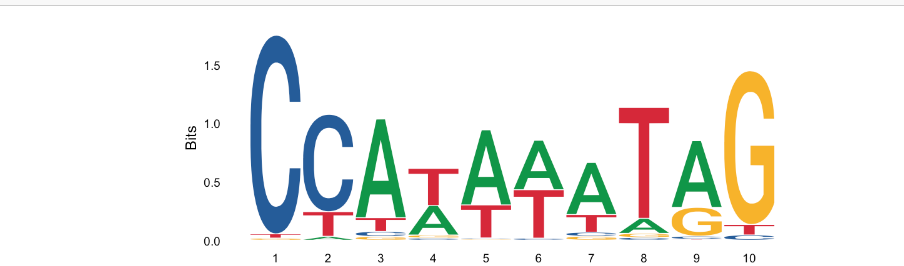添加相关参数： 氨基酸，DNA和RNA序列类型都支持ggseqlogo。默认情况下，ggseqlogo将尝试猜测您的序列类型。您可以通过seq_type选项显式地设置序列类型。

``ggseqlogo( seqs_aa\$AKT1, seq_type='aa' )``

### 以数字的形式展现

``# Replace DNA characters with numbersseqs_numeric = chartr('ATGC','1234', seqs_dna\$MA0001.1)ggseqlogo(seqs_numeric, method='p', namespace=1:4) ``
``# Replace DNA characters with Greek onesseqs_greek = chartr('ATGC', 'δεψλ', seqs_dna\$MA0001.1)ggseqlogo(seqs_greek, namespace='δεψλ', method='p')``

### 颜色的调整

``ggseqlogo(seqs_dna\$MA0001.1, col_scheme='base_pairing')``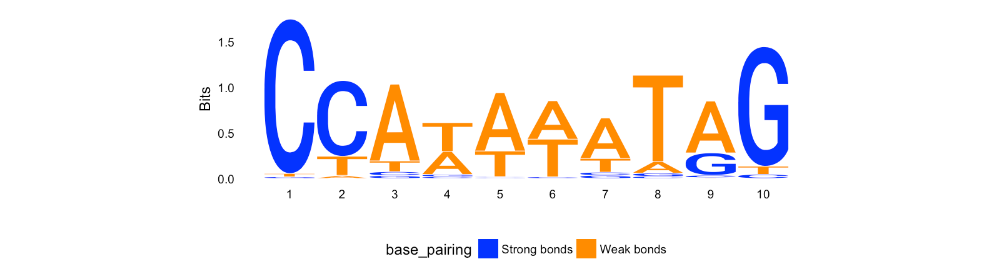`col_scheme`参数有如下几种; `auto`, `chemistry`, `chemistry2`, `hydrophobicity`, `nucleotide`, `nucleotide2`, `base_pairing`, `clustalx`, `taylor`

### 指定颜色

``## 设置颜色cs1 = make_col_scheme(chars=c('A', 'T', 'C', 'G'), groups=c('gr1', 'gr1', 'gr2', 'gr2'),                       cols=c('purple', 'purple', 'blue', 'blue'))## ggseqlogo(seqs_dna\$MA0001.1, col_scheme=cs1)``

### 颜色二

``cs2 = make_col_scheme(chars=c('A', 'T', 'C', 'G'), values=1:4)# Generate sequence logoggseqlogo(seqs_dna\$MA0001.1, col_scheme=cs2)``

### 批量绘制

``ggseqlogo(seqs_dna, ncol=4)## ncol：指定每行的展示个数``

## 自定义高度

``# Create a custom matrix set.seed(123)custom_mat = matrix( rnorm(20), nrow=4, dimnames=list(c('A', 'T', 'G', 'C')))# Generate sequence logoggseqlogo(custom_mat, method='custom', seq_type='dna') + ylab('my custom height')``

1. 最全WGCNA教程（替换数据即可出全部结果与图形）

2. 精美图形绘制教程

3. 转录组分析教程V1